Next: Loop modeling method Up: Methods Previous: Restraints and their derivatives   Contents   Index

# Flowchart of comparative modeling by MODELLER

This section describes a flowchart of comparative modeling by MODELLER, as implemented in the automodel class (see chapter 2).

Input: script file, alignment file, PDB file(s) for template(s).

Output:

 job.log log file job.ini initial conformation for optimization job.rsr restraints file job.sch VTFM schedule file job.B9999???? PDB atom file(s) for the model(s) of the target sequence job.V9999???? violation profiles for the model(s) job.D9999???? progress of optimization job.BL9999???? optional loop model(s) job.DL9999???? progress of optimization for loop model(s) job.IL9999???? initial structures for loop model(s)

The main MODELLER routines used in each step are given in parentheses.

1. Read and check the alignment between the target sequence and the template structures
(alignment.append() and alignment.check()).

2. Calculate restraints on the target from its alignment with the templates:

1. Generate molecular topology for the target sequence (model.generate_topology()). Disulfides in the target are assigned here from the equivalent disulfides in the templates (model.patch_ss_templates()). Any user defined patches are also done here (as defined in the automodel.special_patches() routine).

2. Calculate coordinates for atoms that have equivalent atoms in the templates as an average over all templates (model.transfer_xyz()) (alternatively, read the initial coordinates from a file).

3. Build the remaining unknown coordinates using internal coordinates from the CHARMM topology library (model.build()).

4. Write the initial model to a file with extension .ini (model.write()).

5. Generate stereochemical, homology-derived, and special restraints (Restraints.make()) (alternatively, skip this and assume the restraints file already exists):

 stereochemical restraint_type = 'bond angle dihedral improper' mainchain dihedrals
,
restraint_type = 'phi-psi_binormal' mainchain dihedral
restraint_type = 'omega_dihedral' sidechain dihedral
restraint_type = 'chi1_dihedral' sidechain dihedral
restraint_type = 'chi2_dihedral' sidechain dihedral
restraint_type = 'chi3_dihedral' sidechain dihedral
restraint_type = 'chi4_dihedral' mainchain CA-CA distance restraint_type = 'distance' mainchain N-O distance restraint_type = 'distance' sidechain-mainchain distance restraint_type = 'distance' sidechain-sidechain distance restraint_type = 'distance' ligand distance restraints automodel.nonstd_restraints() routine user defined automodel.special_restraints() routine non-bonded pairs distance restraint_type = 'sphere'; calculated on the fly

6. Write all restraints to a file with extension .rsr (Restraints.write()).

3. Calculate model(s) that satisfy the restraints as well as possible. For each model:

1. Generate the optimization schedule for the variable target function method (VTFM).

2. Read the initial model (usually from the .ini file from 2.d) (model.read()).

3. Randomize the initial structure by adding a random number between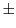automodel.deviation angstroms to all atomic positions (selection.randomize_xyz()).

4. Optimize the model:
• Partially optimize the model by VTFM; Repeat the following steps as many times as specified by the optimization schedule:

• Select only the restraints that operate on the atoms that are close enough in sequence, as specified by the current step of VTFM (Restraints.pick()).
• Optimize the model by conjugate gradients, using only currently selected restraints (conjugate_gradients()).

• Refine the model by simulated annealing with molecular dynamics, if so selected:

5. Calculate the remaining restraint violations and write them out (selection.energy()).

6. Write out the final model to a file with extension .B9999????.pdb where ???? indicates the model number (model.write()). Also write out the violations profile.

7. Superpose the models and the templates, if so selected by automodel.final_malign3d = True, and write them out (alignment.append_model(), alignment.malign3d()).

8. Do loop modeling if so selected using the loopmodel class.Next: Loop modeling method Up: Methods Previous: Restraints and their derivatives   Contents   Index
Automatic builds 2008-10-07